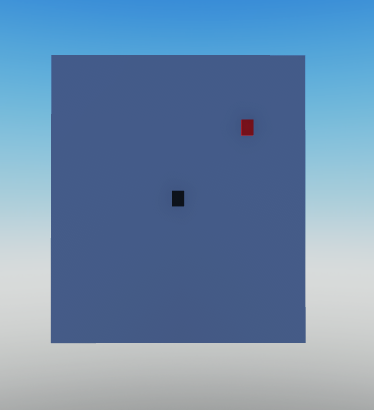# Help Getting Point In World Space From Point On Transformed Plane

My goal is to get a position in world space, from a point relative to an infinite “plane” that is transformed (orientated). I don’t really have or want a plane, but I just use one to visualize it.

Variables I Have:

``````local PlaneOrigin -- The Position of the 'Plane' in world space
local PlaneDirection -- A vector describing its orientation, for context, it is equal to (SomePoint - PlaneOrigin).Unit
local PlanePoint -- A Vector2 relative to the plane
``````

Here is an example of what I want:Figure 1. Plane As 2D, Black Would Be the Origin, Red Is The Dot Wanted

Figure 2. Point Translated To World Space, The Red Arrow Shows the Direction, and Just Like on the 2D Plane, the Red Dot is Whats Wanted

What I have tried:
I tried 2 methods, first one was intuition, but I couldnt get it to work,

``````local localPoint3D = Vector3.new(PlanePoint .X, 0, PlanePoint .Y)
local pointOnPlane = PlaneOrigin + (PlaneDirection * localPoint3D)
``````

The second method got really wonky when approaching 0,

``````local up = PlaneDirection
local right = up:Cross(Vector3.new(PlanePoint .X, 0, PlanePoint .Y)).Unit
local forward = right:Cross(up)
``````

Where the cframe you use it on is the CFrame that represents your plane. So you would go `plane.CFrame:PointToWorldSpace(positionOnPlane) `